# oce

#### 2016-08-12

Abstract. The oce package makes it easy to read, summarize and plot data from a variety of Oceanographic instruments, isolating the researcher from the quirky data formats that are common in this field. It also provides functions for working with basic seawater properties such as the equation of state, and with derived quantities such as the buoyancy frequency. Although simple enough to be used in a teaching context, oce is powerful enough for a research setting. These things are illustrated here in a practical context. Worked exercises are provided to help readers take early steps towards using the oce package in their research.

# 1 Introduction

Oceanographers must deal with measurements made by a wide variety of instruments, a task that is complicated by a tendency of instrument manufacturers to invent new data formats. Although manufacturers often provide software for scanning data files and producing overview plots, this software is of limited use to researchers who work with several instrument types at the same time, and who need to move beyond engineering plots to scientific plots and statistical analysis.

# 2 Architecture of oce objects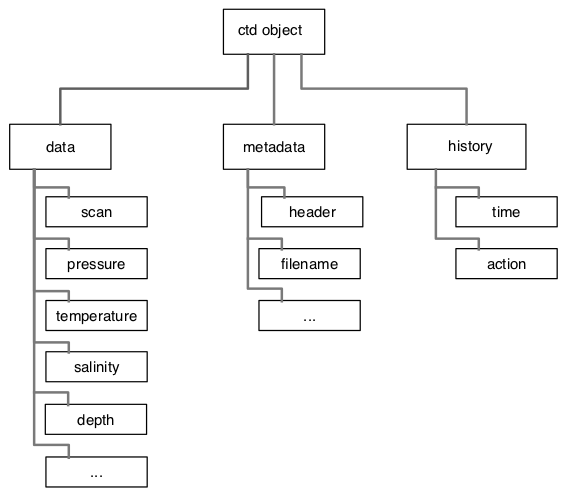Figure 1. Basic layout of a CTD object. All oce objects contain slots named data, metadata, and processingLog, with the contents depending on the type of data.

The need to scan diverse data files was one motivation for the creation of oce, but an equal goal was to make it easy to work with the data once they are in the system. This was accomplished partly by the provision of specialized and generic (overloaded) functions to work with the data, and partly by providing accessor methods that make it convenient to reach inside the data objects (see next section).

As illustrated in Figure 1, each oce object contains three slots:

• data, a list containing the actual data, e.g., for a CTD object, this will contain pressure, temperature, etc.
• metadata, a list containing information about the data, such as units, quality flags, sampling locations, etc.
• processingLog, a list that documents how the object was created and possibly changed thereafter.

# 3 Accessing data in oce objects

For detailed analysis, users may want to access data within oce objects. While it is possible to descend through the object using the “slot” and “list” notation (e.g. d@data$salinity yields the salinity within an oce object named d), this approach is not recommended. It is better to use the [[ oce method, which derives from the generic “Extract” method for accessing parts of an object. To see the full help file, type: ?[[,oce-method For example, to access the salinity field in an oce object, instead of the slot/list notation above, one could type: S <- d[['salinity']] The advantages of the [[ method over directly accessing information in the slots are as follows. 1. It does not require knowledge of where the desired field is contained in the object. (For example, some objects hold latitude in the metadata slot, while others might hold it in the data slot.) 2. It should be familiar to users who understand the basics of R. 3. It permits the extraction of derived values that are stored in the data. For example, if d is a CTD object, then d[["N2"]] will yield the square of the buoyancy frequency (by calling swN2 with reasonable defaults for smoothing parameters). 4. It permits oce to store data compactly, e.g. in 1-byte or 2-byte chunks, instead of using the 8 bytes that are required for numerical data (this permits e.g. working with landsat data in computers with typical memory sizes). # 4 Oce functions The uniformity of the various oce objects helps users build skill in examining and modifying objects. Fine-scale control is provided throughout oce, but the best way to learn is to start with simplest tools and their defaults. For example, the following will read a CTD file named "station1.cnv", summarize the contents, and plot an overview of the data, with profiles, a TS diagram, and a map (Figure 2). library(oce) d <- read.oce("station1.cnv") summary(d) plot(d) The reader should stop now and try this on a file of their own. The pattern will work with a fairly wide variety of file types, because read.oce() examines the file name and contents to try to discover what it is. For an example, if read.oce() is given the name of a file created by an Acoustic Doppler Profiler, it will return an object inheriting from class "adp", so the summary() and plot() calls will be tailored to that type, e.g. the graph will show images of time-distance variation in each of the measured velocity components. Notes on oce function names. 1. As just illustrated, the general function to read a dataset ends in .oce, and the name is a signal that the returned object is of class oce. Depending on the file contents, d will also have an additional class, e.g. if the file contains CTD data, then the object would inherit from two classes, oce and ctd, with the second being used to tailor the graphics by passing control to the ctd variant of the generic plot function (use help("plot,ctd-method") to learn more). 2. Generally, oce functions employ a “camel case” naming convention, in which a function that is described by several words is named by stringing the words together, capitalizing the second and subsequent words. For example, ctdAddColumn() takes a ctd object, and returns a new ctd object that matches the original except for the added column (and an added entry in the processingLog to indicate the addition). 3. Function names begin with oce in cases where a more natural name would be in conflict with a function in the base R system or a package commonly used by Oceanographers. For example, oceContour() is a function that provides an alternative to contour() in the graphics package. # 5 Calculation of seawater properties The oce package provides many functions for dealing with seawater properties. Perhaps the most used is swRho(S,T,p), which computes seawater density $$\rho=\rho(S, T, p)$$, where $$S$$ is salinity, $$T$$ is in-situ temperature in $$^\circ$$C (on the ITS-90 scale), and $$p$$ is seawater pressure, i.e. the excess over atmospheric pressure, in dbar. (This and similar functions starts with the letters sw to designate that they relate to seawater properties.) The result is a number in the order of $$1000$$kg/m$$^3$$. For many purposes, Oceanographers prefer to use the density anomaly $$\sigma=\rho-1000$$kg/m$$^3$$, provided with swSigma(salinity,temperature,pressure), or its adiabatic cousin $$\sigma_\theta$$, provided with swSigmaTheta(). Most of the functions can use either the UNESCO or GSW (TEOS-10) formulation of seawater properties, with the choice set by an argument called eos. It should be noted that oce uses the gsw package for GSW calculations. Caution: the results obtained with eos="gsw" in oce functions may differ from the results obtained when using the gsw functions directly, due to unit conventions. For example, swCSTp(..., eos="gsw") reports conductivity ratio for consistency with the UNESCO formulation, however the underlying gsw function gsw::gsw_C_from_SP() reports conductivity in mS/cm. A partial list of seawater functions is as follows: • swAlpha() for thermal expansion coefficient $$\alpha=-\rho_0^{-1}\partial\rho/\partial T$$ • swAlphaOverBeta() for $$\alpha/\beta$$ • swBeta() for haline compression coefficient $$\beta=\rho_0^{-1}\partial\rho/\partial S$$ • swConductivity() for conductivity from $$S$$, $$T$$ and $$p$$ • swDepth() for depth from $$p$$ and latitude • swDynamicHeight() for dynamic height • swLapseRate() for adiabatic lapse rate • swN2() for buoyancy frequency • swRho() for density $$\rho$$ from $$S$$, $$T$$ and $$p$$ • swSCTp() for salinity from conductivity, temperature and pressure • swSTrho() for salinity from temperature and density • swSigma() for $$\rho-1000$$,kg/m$$^3$$ • swSigmaT() for $$\sigma$$ with $$p$$ set to zero and temperature unaltered • swSigmaTheta() for$$\sigma$$ with $$p$$ set to zero and temperature altered adiabatically • swSoundSpeed() for speed of sound • swSpecificHeat() for specific heat • swSpice() for a quantity used in double-diffusive research • swTFreeze() for freezing temperature • swTSrho() for temperature from salinity and density • swTheta() for potential temperature $$\theta$$ • swViscosity() for viscosity Details and examples are provided in the documentation of these functions. The following exercise may be of help to readers who prefer to learn by doing. (Answers are provided at the end of this document.) Exercise 1. a. What is the density of a seawater parcel at pressure 100 dbar, with salinity 34 PSU and temperature 10$$^\circ$$C? b. What temperature would the parcel have if raised adiabatically to the surface? c. What density would it have if raised adiabatically to the surface? d. What density would it have if lowered about 100m, increasing the pressure to 200 dbar? e. Draw a blank $$T$$-$$S$$ diagram with $$S$$ from 30 to 40 PSU and $$T$$ from -2 to 20$$^\circ$$C. # 6 CTD data ## 6.1 Example with pre-trimmed data Many of the object types supported by oce come with built-in data. For an example, data(ctd) yields a CTD profile that has been trimmed to just the downcast portion of the sampling. (See the next section to learn how to do this trimming.) A summary and plot (Figure 2) are created as follows. library(oce) data(ctd) summary(ctd) #> CTD Summary #> ----------- #> #> * Instrument: SBE #> * Instr. serial no.: #> * Temp. serial no.: 1140 #> * Cond. serial no.: 832 #> * File: /Users/kelley/src/oce/create_data/ctd/ctd.cnv #> * Original file: c:\seasoft3\basin\bed0302.hex #> * Institute: #> * Chief scientist: #> * Date: 2003-10-15 11:38:38 #> * Start time: 2003-10-15 11:38:38 #> * System upload time: 2003-10-15 11:38:38 #> * Cruise: Halifax Harbour #> * Vessel: Divcom3 #> * Station: Stn 2 #> * Deployment type: unknown #> * Location: 44.684N 63.644W #> * Water depth: NA #> * Statistics of data #>  #> Min. Mean Max. Dim. OriginalName #> scan 130 220 310 181 scan #> time [s, elapsed] 129 219 309 181 timeS #> pressure [dbar] 1.48 22.885 44.141 181 pr #> depth [m] 1.468 22.698 43.778 181 depS #> temperature [°C, IPTS-68] 2.919 7.5063 14.237 181 t068 #> salinity [PSS-78] 29.916 31.219 31.498 181 sal00 #> flag 0 0 0 181 flag #>  #> * Processing Log #> - 2016-07-24 11:26:05.173 UTC: create 'ctd' object #> - 2016-07-24 11:26:05.218 UTC: read.ctd.sbe(file = file, processingLog = processingLog) #> - 2016-07-24 11:26:05.277 UTC: oce.edit(x = ctd, item = "startTime", value = ctd[["systemUploadTime"]], reason = "startTime is not in file", person = "Dan Kelley") plot(ctd)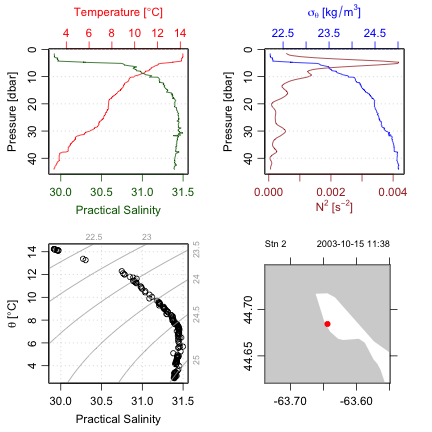Figure 2. An overview of a ctd dataset. Accessing the data within this ctd object can be done directly, e.g. ctd@data$pressure holds the pressure record, but it is usually better to use an accessor function that is provided with oce. This function is named [[, and it takes a character string as an argument, e.g. ctd[["pressure"]] yields the pressure column. The accessor notation is preferable to direct access because it is simpler for the user. For example, several oce objects store the data in single-byte or two-byte chunks, to match the raw format used by the instruments, and the accessor function takes care of translating these values to what are sometimes called “science” units.

Exercise 2. Plot a profile of $$\sigma_\theta$$ and $$N^2$$, for the data in the pycnocline. (Hint: use subset().)

## 6.2 Example with raw data

Practicing Oceanographers may be wondering how the CTD cast used in the preceding section was trimmed of equilibration-phase and upcast-phase data. Spurious data from these phases must be trimmed as a first step in processing. For example, consider the following code.

data(ctdRaw)
plotScan(ctdRaw)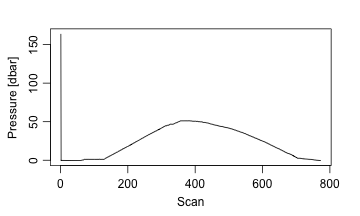Figure 3. Scanwise plot of the ctdRaw sample data set. Note the spike at the start, the equilibration phase before the downcast, and the spurious freshening signal near the start of the upcast.

This produces a two-panel plot (Figure 3) of the data as a time-series, revealing not just the desired downcast, but also an earlier equilibration phase and a later upcast. The x-axis in Figure 3 is the scan number, which is a convenient index for extraction of the downcast portion of the profile by an essentially manual method, e.g. proceeding with a sequence of commands such as

plotScan(ctdTrim(ctdRaw, "range",
parameters=list(item="scan", from=140, to=250)))
plotScan(ctdTrim(ctdRaw, "range",
parameters=list(item="scan", from=150, to=250)))

This method of making decisions based on plotted information is probably the most robust method of trimming data. However, for quick work, users may be satisfied with the results of automatic downcast detection, e.g.

ctdTrimmed <- ctdTrim(ctdRaw)

It should be noted that ctdTrim() inserts entries into the object’s log file, so that the details of how the trimming was done are recorded together with the data.

Once the profile has been trimmed, one may wish to use ctd.decimate() to smooth the data and interpolate the smoothed results to uniformly-spaced pressure values.

Taking these things together, a quick visual examination of a CTD file takes just one line of code:

plot(ctdDecimate(ctdTrim(read.ctd("stn123.cnv"))))

## 6.3 Example with WOCE archive data

The package has a harder time scanning the headers of data files in the WOCE archive format than it does in the Seabird format illustrated in the previous examples. This is mainly because front-line researchers tend to work in the Seabird format, and partly because the WOCE format is odd. For example, the first line of a WOCE file is of the form CTD,20060609WHPOSIODAM (or BOTTLE,...). Scanning the item to the left of the comma is not difficult (although there are variants to the two shown, e.g. CTDO sometimes occurs). The part to the right of the comma is more difficult. The first part is a date (yyyymmdd) so that is no problem. But then things start to get tricky. In the example provided, this text contains the division of the institute (WHPO), the institute itself (SIO), and initial of the investigator (DAM). The problem is that no dividers separate these items, and that there seem to be no standards for the item lengths. Rather than spend a great deal of time coding special cases (e.g. scanning to see if the string WHOI occurs in the header line), the approach taken with oce is to ignore such issues relating to quirky headers, on the assumption that users can scan human-written headers with high skill.

Quite commonly, CTD profiles taken during a cruise are collected together in a sequence of files in a given directory. For a real-world example, one might visit the website mentioned in the code provided below, download and expand the zip file, enter the directory thus formed, and run the code to get an overall TS plot for all the CTD stations of this cruise. (Caution: the link seems to change from time to time.)

library(oce)
# http://cchdo.ucsd.edu/data/7971/ar18_58JH19941029_ct1.zip
files <- system("ls *.csv", intern=TRUE)
for (i in 1:length(files)) {
if (i == 1) {
plotTS(x, Slim=c(31, 35.5), Tlim=c(-2, 10), type='o')
} else {
points(x[["salinity"]], x[["potential temperature"]])
lines(x[["salinity"]], x[["potential temperature"]])
}
}

In the above, lines connect the points within a given profile. This can be a useful method for a quick scan looking for outliers. Another is to colour-code the profiles, although this gets confusing with large datasets, in which case the method of the following exercise might be useful.

Exercise 3. (advanced) Make a multi-file plot summarizing the TS relationship, with each file showing the overall relationship in gray and the individual profile in black.

# 7 Section plots

The commands

data(section)
plot(section, which=c(1, 2, 3, 99))

will plot a summary diagram containing sections of $$T$$, $$S$$, and $$\sigma_\theta$$, along with a chart indicating station locations. In addition to such overview diagrams, the section variant of the generic plot function can also create individual plots of individual properties (use help("plot,section-method") to learn more).

Some section datasets are supplied in a pre-gridded format, but it is also common to have different pressure levels at each station. For such cases, sectionGrid() may be used, e.g. the following produces Figure 4. The ship was travelling westward from the Mediterranean towards North America, taking 124 stations in total; the stationId value selects the last few stations of the section, during which the ship headed toward the northwest, crossing isobaths (and perhaps, the Gulf Stream) at nearly right angles.

library(oce)
data(section)
GS <- subset(section, 102 <= stationId & stationId <= 124)
GSg <- sectionGrid(GS, p=seq(0, 1600, 25))
plot(GSg, which=c(1,99), map.xlim=c(-85,-(64+13/60)))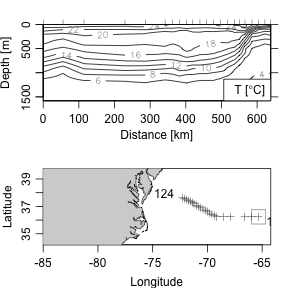Figure 4. Portion of the CTD section designated A03, showing the Gulf Sream region. The square on the cruise track corresponds to zero distance on the section.

Exercise 4. Draw a $$T$$-$$S$$ diagram for the section data, using black symbols to the east of 30W and gray symbols to the west, thus highlighting Mediterranean-derived waters. (Hint: use the accessor function [[.)

Exercise 5. Plot dynamic height and geostrophic velocity across the Gulf Stream. (Hint: use the swDynamicHeight() function.)

# 8 Maps

There are several ways to handle map projections in R and other systems. Oce uses the rgdal package to calculate the details of map projections. A wide group of functions is provided for plotting maps. The first step is to call mapPlot() to construct the map, after which points can be added with mapPoints(), lines with mapLines(), etc. For example, the following plots a world coastline in the Winkel Tripel projection, with the cruise track for the section dataset in red.

library(oce)
data(coastlineWorld)
par(mar=rep(0, 4))
mapPlot(coastlineWorld, projection="+proj=wintri", col="lightgray")
data(section)
lon <- section[["longitude", "byStation"]]
lat <- section[["latitude", "byStation"]]
mapLines(lon, lat, col='red', cex=0.5)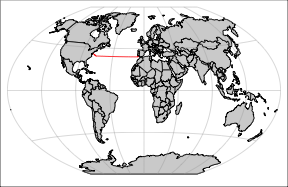Figure 5. World in Winkel Tripel projection, with the section profile sites indicated.

There are far too many projections to illustrate here. See http://dankelley.github.io/r/2015/04/03/oce-proj.html for a blog item that provides examples of the available projections. Note that some have a problem with spurious horizontal lines. This can result from coastline segments that cross the edge of the plotting area, and getting rid of this is tricky enough to be the heart of the longest-lived bug in the oce issue list, i.e. https://github.com/dankelley/oce/issues/388. In some instances the function coastlineCut() can help, but it is provisional and subject to change.

# 9 Sea-level data

## 9.1 Time-domain analysis

The following code graphs a built-in dataset of sea-level time series (Figure 9). The top panel provides an overview of the entire data set. The second panel is narrowed to the most recent month, which should reveal spring-neap cycles if the tide is mixed. The third panel is a log spectrum, with a few tidal constituents indicated. The section variant of the generic plot function provides other possibilities for plotting, including a cumulative spectrum that can be quite informative (use help("plot,sealevel-method") to learn more).

library(oce)
data(sealevel)
plot(sealevel)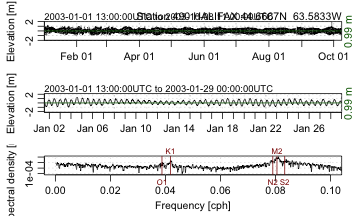Figure 9. Sea-level timeseries measured in 2003 in Halifax Harbour.

Exercise 6. Illustrate Halifax sea-level variations during Hurricane Juan, near the end of September, 2003.

## 9.2 Tidal-harmonic analysis

A preliminary version of tidal analysis is provided by the tidem function provided in this version of the package, but readers are cautioned that the results are certain to change in a future version. (The problems involve phase and the inference of satellite nodes.)

Exercise 7. Plot the de-tided Halifax sea level during Autumn 2003, to see whether Hurricane Juan is visible. (Hint: use predict with the results from tidem.)

# 10 Acoustic Doppler Profiler data

The following commands produce Figure 10, showing one velocity component of currents measured in the St Lawrence Estuary Internal Wave Experiment. This plot type is just one of many provided by the adp variant of the generic plot function (use help("plot,adp-method") to learn more).

library(oce)
lines(adp[['time']], adp[['pressure']], lwd=2)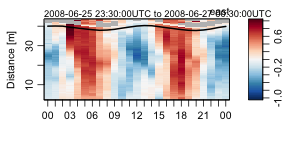Figure 10. Measurements made with a bottom-mounted ADP in the St Lawrence Estuary. The line near the surface indicates pressure measured by the ADP.

# 11 Handling data-quality flags

Hydrographic and some other data may be accompanied by data-quality flags. For example, salinity is subject to a variety of instrumental and other errors, and so archives of its value commonly contain quality flags. These flags may derive from statistical checks, from the judgement of a human analyst, or a combination of the two.

Some flags are in the form of numerical values (typically integers) but others may be expressed as character strings. A frustrating aspect of oceanographic analysis is that different data archiving agencies employ different systems for flags. For example, the World Hydrographic Programme designates good bottle/CTD data with a flag value of 2, whereas the World Ocean Database uses 0 for good data. It is also common to indicate bad data by setting values to non-physical values, e.g. -999, -99.99, or similar, and sometimes these coded values may contradict more formal flags, when both are present.

Dealing with flags often requires ad hoc analysis methods, and for this reason, the oce package offers tools for dealing with flags in a variety of ways. The first thing to realize is that only certain data types contain flags, and only certain data objects will contain flags. For example, hydrographic data are quite likely to be provided with data-quality flags, but these are never provided with coastline data.

Data-quality flags are stored in the flags entry in the metadata slot of oce objects. This entry is a list, containing items with names corresponding to names of data elements that are stored in the data slot. Direct manipulation is possible, although it is more convenient to use the [[ and [[<- access methods provided in oce, or, for some tasks, the handleFlags function provided by oce.

## 11.2 Retrieving and changing flags

An example should clarify a working procedure. The section dataset provided with oce holds many ctd objects that each have data-quality flags. The first few salinity flags for the 100th station may be found as follows.

data(section)
stn <- section[["station", 100]]
#>  2 2 2 2 2 3

Note that these data use the WHP scheme, i.e. good data are indicated with 2, whereas questionable data are indicated with 3.

Before discussing how such flags might be handled, it is important to note that it is often necessary to alter such flags. For example, some datasets will have flags set to indicate good data, even though the data are set to -999, which is one of several old-fashioned missing-value codes. The section dataset does not contain any such cases, but the situation may be simulated as follows.

# fake second datum
stn[["salinity"]] <- -999

With this, the setting of flags might be done with

stn[["salinityFlag"]] <- ifelse(stn[["salinity"]] < 0, 3, stn[["salinityFlag"]])

with a check being done with

head(stn[["salinityFlag"]])
#>  2 3 2 2 2 3

It should be obvious to the reader that this procedure could be extended in more sophisticated ways, e.g. with schemes searching for pressure inversions, large departures from a TS relationship, loops on TS diagrams, etc. It can be useful to combine automatic schemes with interactive plotting, to identify bad data.

Exercise 8. Manipulating flags. The dataset provided with oce using

library(oce)
d <- read.oce(system.file("extdata", "d201211_0011.cnv", package="oce"))

contains a column named flags that consists of a 3-digit number. THe first digit is a flag for temperature, the second for conductivity, and the third for oxygen. Compute the individual flags and add them to the metdata of the ctd object.

## 11.3 Using flags to manipulate data

A conservative approach is to set data to the missing-value code NA if the data-quality flag indicates a problem. For example, in the WHP scheme, values other than 2 indicate unchecked, bad or suspect data, and those data could be set to the missing-value code as follows, where stn2 is the new version:

stn2 <- stn
stn2[["salinity"]] <- ifelse(stn2[["salinityFlag"]]!=2, NA, stn2[["salinity"]])
#>  2 3 2 2 2 3

This can be expressed more compactly with handleFlags, e.g.

stn3 <- stn
stn3[["salinity"]] <- -999
stn3 <- handleFlags(stn3, list(salinity=c(1,3:9)))

A summary of the salinities follows

head(data.frame(stnS=stn[["salinity"]], stn2S=stn2[["salinity"]], stn3S=stn3[["salinity"]]))
#>        stnS   stn2S   stn3S
#> 1   36.4766 36.4766 36.4766
#> 2 -999.0000      NA      NA
#> 3   36.6001 36.6001 36.6001
#> 4   36.5399 36.5399 36.5399
#> 5   36.2388 36.2388 36.2388
#> 6   35.7580      NA      NA

# 12 Working in non-English languages

Many of the oce plotting functions produce axis labels that can be displayed in languages other than English. At the time of writing, French, German, Spanish, and Mandarin are supported in at least a rudimentary way. Setting the language can be done at the general system level, or within R, as indicated below (results not shown).

library(oce)
Sys.setenv(LANGUAGE="fr")
data(ctd)
plot(ctd)

Most of the translated items were found by online dictionaries, and so they may be incorrect for oceanographic usage. Readers can help out in the translation effort, if they have knowledge of how nautical words such as Pitch and Roll and technical terms such as Absolute Salinity and Potential Temperature should be written in various languages.

# 13 The future of oce

The present version of oce can only handle data types that the authors have been using in their research. New data types will be added as the need arises in that work, but the authors would also be happy to add other data types that are likely to prove useful to the Oceanographic community. (The data types need not be restricted to Physical Oceanography, but the authors will need some help in dealing with other types of data, given their research focus.)

Two principles will guide the addition of data types and functions: (a) the need, as perceived by the authors or by other contributors and (b) the ease with which the additions can be made. One might call this development-by-triage, by analogy to the scheme used in Emergency Rooms to organize medical effort efficiently.

# 14 Development website

The site http://github.com/dankelley/oce provides a window on the development that goes on between the CRAN releases of the package. Readers are requested to visit the site to report bugs, to suggest new features, or just to see how oce development is coming along. Note that the development branch is used by the authors in their work, and is updated so frequently that it must be considered unstable, at least in those spots being changed on a given day. Official CRAN releases derive from the master branch, and are done when the code is considered to be of reasonable stability and quality. This is all in a common pattern for open-source software.

Exercise 1. Seawater properties.

library(oce)
swRho(34, 10, 100)
#>  1026.624
swTheta(34, 10, 100)
#>  9.990996
swRho(34, swTheta(34, 10, 100), 0)
#>  1026.173
swRho(34, swTheta(34, 10, 100, 200), 200)
#>  1027.073
plotTS(as.ctd(c(30,40),c(-2,20),rep(0,2)), grid=TRUE, col="white")

Exercise 2. Plot a profile of $$\sigma_\theta$$ and $$N^2$$, for the data in the pycnocline. (Hint: use subset().)

Although one may argue as to the limits of the pycnocline, for illustration let us say it is in 5 dbar to 12 dbar range. One way to do this is

library(oce)
data(ctd)
pycnocline <- ctdTrim(ctd, "range",
parameters=list(item="pressure", from=5, to=12))
plotProfile(pycnocline, which="density+N2")

Another is

library(oce)
data(ctd)
pycnocline <- subset(ctd, 5 <= pressure & pressure <= 12)
plotProfile(pycnocline, which="density+N2")

Exercise 3. Make a multi-file plot summarizing the TS relationship, with each file showing the overall relationship in gray and the individual profile in black. (This is an advanced exercise requiring some R skills.)

The code provided below creates 91 PNG files, with names ar18_01.png, ar18_02.png, etc. Loading these in a view that permits quick paging through this file list is an easy way to spot suspicious data, since each plot has the station number at the top. (Users trying this example should bear in mind that this is a fairly large dataset, so the processing will take up to a minute.)

library(oce)
# http://cchdo.ucsd.edu/data/7971/ar18_58JH19941029_ct1.zip
files <- system("ls *.csv", intern=TRUE)
n <- length(files)
ctds <- vector("list", n) # to hold the CTD objects
station <- vector("list", n)
for (i in 1:n) {
station[[i]] <- ctds[[i]][["station"]]
}
S <- unlist(lapply(1:n, function(i) ctds[[i]][["salinity"]]))
T <- unlist(lapply(1:n, function(i) ctds[[i]][["temperature"]]))
p <- unlist(lapply(1:n, function(i) ctds[[i]][["pressure"]]))
overall <- as.ctd(S, T, p)
png("ar18_%02d.png")
for (i in 1:n) {
plotTS(overall, col='gray')
lines(ctds[[i]][["salinity"]], ctds[[i]][["potential temperature"]])
mtext(station[i], side=3, line=0)
}
dev.off()

Exercise 4. Draw a $$T$$-$$S$$ diagram for the section data, using black symbols to the east of 30W and gray symbols to the west, thus highlighting Mediterranean-derived waters. (Hint: use the accessor function [[.)

library(oce)
data(section)
ctd <- as.ctd(section[["salinity"]], section[["temperature"]], section[["pressure"]])
col <- ifelse(section[["longitude"]] > -30, "black", "gray")
plotTS(ctd, col=col)

Exercise 5. Plot dynamic height and geostrophic velocity across the Gulf Stream. (Hint: use the swDynamicHeight function.)

(Try ?swDynamicHeight for hints on smoothing.)

library(oce)
data(section)
GS <- subset(section, 102 <= stationId & stationId <= 124)
dh <- swDynamicHeight(GS)
par(mfrow=c(2,1), mar=c(3, 3, 1, 1), mgp=c(2, 0.7, 0))
plot(dh$distance, dh$height, type="l", xlab="", ylab="Dyn. Height [m]")
grid()
# 1e3 metres per kilometre
latMean <- mean(GS[["latitude"]])
f <- coriolis(latMean)
g <- gravity(latMean)
v <- diff(dh$height)/diff(dh$distance) * g / f / 1e3
plot(dh$distance[-1], v, type="l", xlab="Distance [km]", ylab="Velocity [m/s]") grid() abline(h=0, col='red') Exercise 6. Halifax sea-level during Hurricane Juan, near the end of September, 2003. A web search will tell you that Hurricane Juan hit about midnight, 2003-sep-28. The first author can verify that the strongest winds occurred a bit after midnight – that was the time he moved to a room without windows, in fear of flying glass. library(oce) data(sealevel) # Focus on 2003-Sep-28 to 29th, the time when Hurricane Juan caused flooding plot(sealevel,which=1,xlim=as.POSIXct(c("2003-09-24","2003-10-05"), tz="UTC")) abline(v=as.POSIXct("2003-09-29 04:00:00", tz="UTC"), col="red") mtext("Juan", at=as.POSIXct("2003-09-29 04:00:00", tz="UTC"), col="red") Exercise 7. Plot the de-tided Halifax sea level during Autumn 2003, to see whether Hurricane Juan is visible. (Hint: use predict with the results from tidem.) library(oce) data(sealevel) m <- tidem(sealevel) oce.plot.ts(sealevel[['time']], sealevel[['elevation']] - predict(m), ylab="Detided sealevel [m]", xlim=c(as.POSIXct("2003-09-20"), as.POSIXct("2003-10-08"))) The spike reveals a surge of about 1.5m, on the 29th of September, 2003. Exercise 8. Manipulating flags. library(oce) d <- read.oce(system.file("extdata", "d201211_0011.cnv", package="oce")) ## The following is in the .cnv file: # Processing Notes: Flag word has 3 columns: Temperature, Conductivity, and Oxygen # Processing Notes: Flag_code: 0 = no QC; 2 = good; 6 = interpolated, or replaced by dual sensor or upcast value; flag <- d[['flag']] flag1 <- floor(flag/100) flag2 <- floor((flag-100*flag1)/10) flag3 <- floor((flag-100*flag1-10*flag2)) d[["flags"]]$temperature <- flag1
d[["flags"]]$conductivity <- flag2 d[["flags"]]$oxygen <- flag3